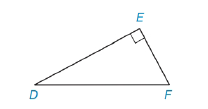Chapter 5.4, Problem 10EElementary Geometry For College St...

7th Edition
Alexander + 2 others
ISBN: 9781337614085

Solutions

Chapter
SectionElementary Geometry For College St...

7th Edition
Alexander + 2 others
ISBN: 9781337614085
Textbook Problem

Find DF if:a) D E = 12 and E F = 5 b) D F = 12 and E F = 6 .Exercises 7-10

To determine

(a)

To Find:

The length of DF¯.

Explanation

Given DE=12 and EF= 5,

And figure,

From the figure,

ΔDEF is a right triangle with right angle E

From the Pythagorean Theorem,

The square of the length of hypotenuse of a right triangle is equal to the sum of the square of the lengths of the other two sides.

From the figure, DF¯ is the hypotenuse,

DF2=E<

To determine

(b)

To Find:

The length of DF¯.

Still sussing out bartleby?

Check out a sample textbook solution.

See a sample solution

The Solution to Your Study Problems

Bartleby provides explanations to thousands of textbook problems written by our experts, many with advanced degrees!

Get Started

In Exercises 1-6, simplify the expression. 5. (4x1)(3)(3x+1)(4)(4x1)2

Applied Calculus for the Managerial, Life, and Social Sciences: A Brief Approach

Find the limit. limx1(1x1+1x23x+2)

Calculus (MindTap Course List)

Estimate 14(x3x2) using the Left Endpoint Rule and n = 5. a) 203 b) 20 c) 24 d) 30

Study Guide for Stewart's Single Variable Calculus: Early Transcendentals, 8th

What are the five steps of the scientific method?

Research Methods for the Behavioral Sciences (MindTap Course List)